# Robust Vibration Control in Flexible Beam

This example shows how to robustly tune a controller for reducing vibrations in a flexible beam. This example is adapted from "Control System Design" by G. Goodwin, S. Graebe, and M. Salgado.

### Uncertain Model of Flexible Beam

Figure 1 depicts an active vibration control system for a flexible beam.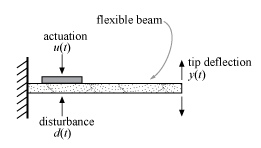Figure 1: Active control of flexible beam

In this setup, a sensor measures the tip position $y\left(t\right)$ and the actuator is a piezoelectric patch delivering a force $u\left(t\right)$. We can model the transfer function from control input $u$ to tip position $y$ using finite-element analysis. Keeping only the first six modes, we obtain a plant model of the form

`$G\left(s\right)=\sum _{i=1}^{6}\frac{{\alpha }_{i}}{{s}^{2}+2{\zeta }_{i}{\omega }_{i}s+{\omega }_{i}^{2}}$`

with the following nominal values for the amplitudes :

`$\alpha =9.72×1{0}^{-4},0.0122,0.0012,-0.0583,-0.0013,0.1199$`

`$\omega =18.95,118.76,332.54,651.66,1077.2,1609.2.$`

The damping factors ${\zeta }_{i}$ are often poorly known and are assumed to range between 0.0002 and 0.02. Similarly, the natural frequencies are only approximately known and we assume 20% uncertainty on their location. To construct an uncertain model of the flexible beam, use the `ureal` object to specify the uncertainty range for the damping and natural frequencies. To simplify, we assume that all modes have the same damping factor $\zeta$.

```% Damping factor zeta = ureal('zeta',0.002,'Range',[0.0002,0.02]); % Natural frequencies w1 = ureal('w1',18.95,'Percent',20); w2 = ureal('w2',118.76,'Percent',20); w3 = ureal('w3',332.54,'Percent',20); w4 = ureal('w4',651.66,'Percent',20); w5 = ureal('w5',1077.2,'Percent',20); w6 = ureal('w6',1609.2,'Percent',20);```

Next combine these uncertain coefficients into the expression for $G\left(s\right)$.

```alpha = [9.72e-4 0.0122 0.0012 -0.0583 -0.0013 0.1199]; G = tf(alpha(1),[1 2*zeta*w1 w1^2]) + tf(alpha(2),[1 2*zeta*w2 w2^2]) + ... tf(alpha(3),[1 2*zeta*w3 w3^2]) + tf(alpha(4),[1 2*zeta*w4 w4^2]) + ... tf(alpha(5),[1 2*zeta*w5 w5^2]) + tf(alpha(6),[1 2*zeta*w6 w6^2]); G.InputName = 'uG'; G.OutputName = 'y';```

Visualize the impact of uncertainty on the transfer function from $u$ to $y$. The `bode` function automatically shows the responses for 20 randomly selected values of the uncertain parameters.

```rng(0), bode(G,{1e0,1e4}), grid title('Uncertain beam model')```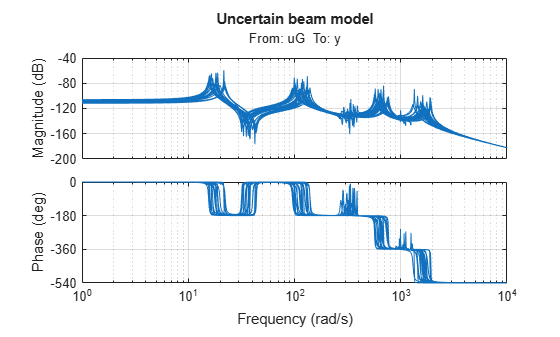### Robust LQG Control

LQG control is a natural formulation for active vibration control. With `systune`, you are not limited to a full-order optimal LQG controller and can tune controllers of any order. Here for example, let's tune a 6th-order state-space controller (half the plant order).

`C = tunableSS('C',6,1,1);`

The LQG control setup is depicted in Figure 2. The signals $d$ and $n$ are the process and measurement noise, respectively.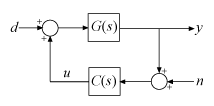Figure 2: LQG control structure

Build a closed-loop model of the block diagram in Figure 2.

```C.InputName = 'yn'; C.OutputName = 'u'; S1 = sumblk('yn = y + n'); S2 = sumblk('uG = u + d'); CL0 = connect(G,C,S1,S2,{'d','n'},{'y','u'});```

Note that `CL0` depends on both the tunable controller `C` and the uncertain damping and natural frequencies.

`CL0`
```CL0 = Generalized continuous-time state-space model with 2 outputs, 2 inputs, 18 states, and the following blocks: C: Parametric 1x1 state-space model, 6 states, 1 occurrences. w1: Uncertain real, nominal = 18.9, variability = [-20,20]%, 3 occurrences w2: Uncertain real, nominal = 119, variability = [-20,20]%, 3 occurrences w3: Uncertain real, nominal = 333, variability = [-20,20]%, 3 occurrences w4: Uncertain real, nominal = 652, variability = [-20,20]%, 3 occurrences w5: Uncertain real, nominal = 1.08e+03, variability = [-20,20]%, 3 occurrences w6: Uncertain real, nominal = 1.61e+03, variability = [-20,20]%, 3 occurrences zeta: Uncertain real, nominal = 0.002, range = [0.0002,0.02], 6 occurrences Type "ss(CL0)" to see the current value, "get(CL0)" to see all properties, and "CL0.Blocks" to interact with the blocks. ```

Use an LQG criterion as control objective. This tuning goal lets you specify the noise covariances and the weights on the performance variables.

`R = TuningGoal.LQG({'d','n'},{'y','u'},diag([1,1e-10]),diag([1 1e-12]));`

Now tune the controller `C` to minimize the LQG cost over the entire uncertainty range.

`[CL,fSoft,~,Info] = systune(CL0,R);`
```Soft: [5.63e-05,Inf], Hard: [-Inf,Inf], Iterations = 75 Soft: [6.26e-05,0.000121], Hard: [-Inf,-Inf], Iterations = 114 Soft: [6.96e-05,7.36e-05], Hard: [-Inf,-Inf], Iterations = 120 Soft: [7.19e-05,7.19e-05], Hard: [-Inf,-Inf], Iterations = 46 Final: Soft = 7.19e-05, Hard = -Inf, Iterations = 355 ```

### Validation

Compare the open- and closed-loop Bode responses from $d$ to $y$ for 20 randomly chosen values of the uncertain parameters. Note how the controller clips the first three peaks in the Bode response.

```Tdy = getIOTransfer(CL,'d','y'); bode(G,Tdy,{1e0,1e4}) title('Transfer from disturbance to tip position') legend('Open loop','Closed loop')```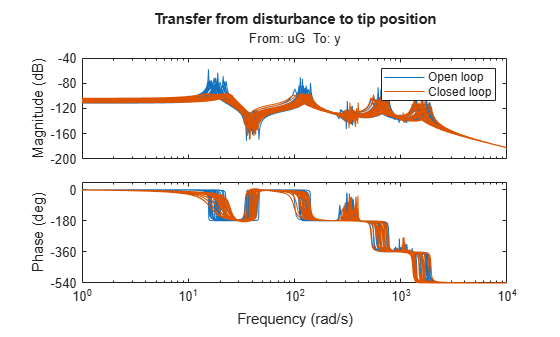Next plot the open- and closed-loop responses to an impulse disturbance $d$. For readability, the open-loop response is plotted only for the nominal plant.

```impulse(getNominal(G),Tdy,5) title('Response to impulse disturbance d') legend('Open loop','Closed loop')```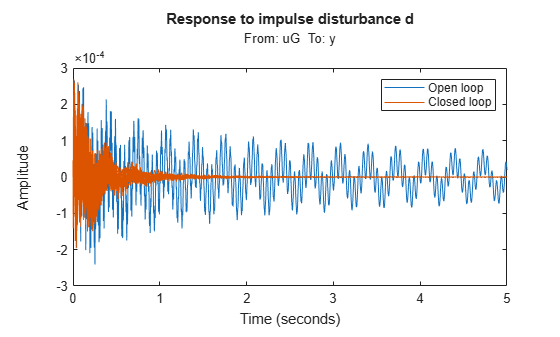Finally, `systune` also provides insight into the worst-case combinations of damping and natural frequency values. This information is available in the output argument `Info`.

`WCU = Info.wcPert`
```WCU=3×1 struct array with fields: w1 w2 w3 w4 w5 w6 zeta ```

Use this data to plot the impulse response for the two worst-case scenarios.

```impulse(usubs(Tdy,WCU),5) title('Worst-case response to impulse disturbance d')```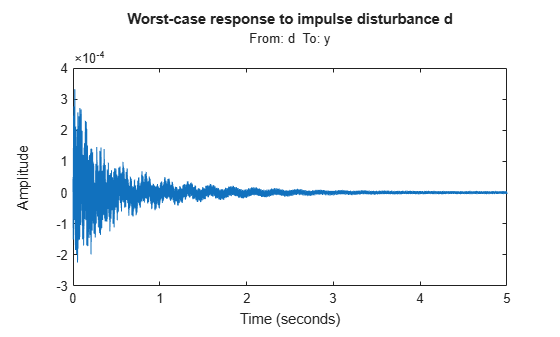##### Support평가판 신청